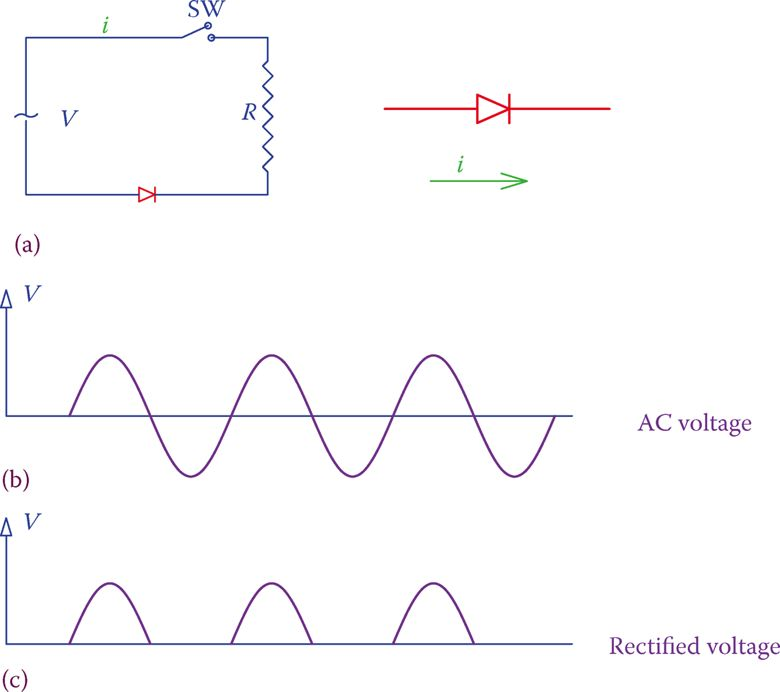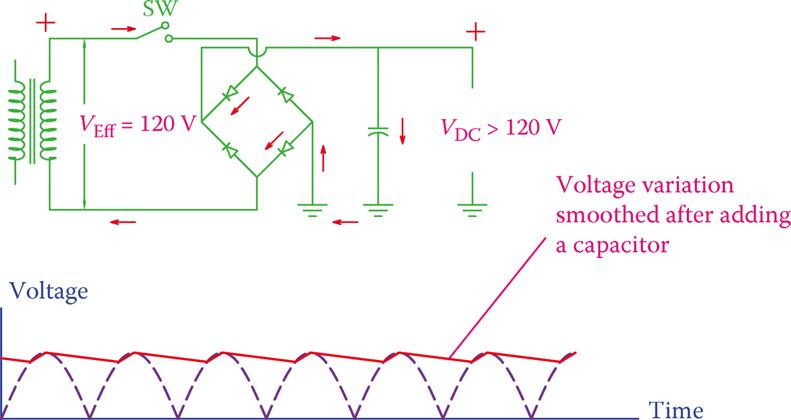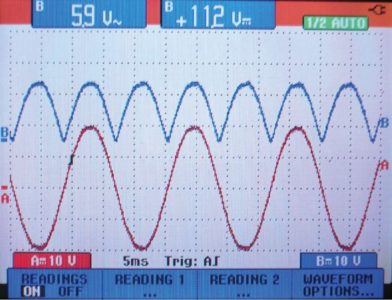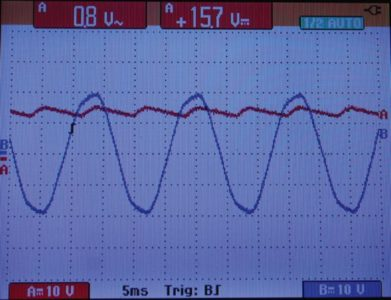# Half Wave & Full Wave Rectifier | Working Principle | Circuit Diagram

Want create site? Find Free WordPress Themes and plugins.

A rectifier is a device to convert AC electricity to DC. Except for batteries that are small sources of DC electricity, or the rechargeable ones that store electricity, most of the domestic devices that work with DC electricity use rectifiers.

At the industrial level, there are industries that need DC electricity to run DC motors or processes that can function only with DC; they either must have their own generators or must obtain their DC requirement from AC sources by rectifiers.

Both single-phase and three-phase AC can be converted to DC. For domestic products and small applications, single-phase rectifiers suffice, but for large loads at the industry level, such as electroplating, electrolytic metal refining, and high voltage direct current (HVDC) transmission and smaller ones like DC motor drives, three-phase converters are employed.

The converter is a term that is used for both rectifier and inverter (inverter does the opposite job of providing AC from DC.

In the simplest form a rectifier consists of a number of diodes, and therefore, we can call it a diode rectifier. Diode rectifiers are simpler than the other types that use switching devices. The most common and widely used single-phase rectifier is the bridge rectifier, but full-wave rectifiers and half-wave rectifiers can also be used.

Bridge rectifier: Full-wave rectifier of AC using four diodes (for single phase) or six diodes (for three phase) to obtain direct current from alternating current.

Full-wave rectifier: Rectifier in which both half cycles of an alternating current waveform are rectified and delivered to the output as DC, as opposed to a half-wave rectifier in which only one half of each cycle reaches the output.

Half-wave rectifier: Simplest type of rectifier for alternating current, consisting of only one diode (for single phase) and three diodes (for three-phase) that block the negative half cycle of AC, so that only the positive half cycles are passing to the output.

## Half-Wave Rectifier

Figure 1 shows the schematic of a half-wave rectifier, which is the simplest and lowest quality type of rectifier. But, it demonstrates the principle of how rectifiers work.

It consists of only one diode inserted in an AC circuit. As a result, for each full cycle of the alternating current, the diode conducts only for half of the cycle but blocks the current for the other half. The outcome is shown in Figure 1c, which is a DC voltage as seen by a load.

The performance of a half-wave rectifier is very poor, and the DC voltage has a lot of variation. The DC voltage here is, in fact, a series of half sinusoidal pulses (a pulse is a short duration DC signal). This variation of the voltage level, called a ripple, can be smoothed out to some extent by employing a filter.

Note that the variation of voltage reflects in a load, depending on what the load consists of. Unless otherwise said, the load for this rectifier is everything that is connected in the circuit and is represented by R in Figure 1.

Ripple: Fluctuations in a rectified AC waveform. Fast fluctuations in an electrical value, such as voltage, that is supposed to be constant.Figure 1 Half-wave rectifier. (a) AC circuit. (b) AC voltage across resistor without the diode. (c) The voltage across the resistor when a diode is added to the circuit.Figure 2 Circuit Diagram of a half-wave rectifier and the effect of a filter on the output voltage.

The most common filter is one or more capacitors connected across the positive and negative poles of the DC voltage, that is, in parallel with the load.

Half-wave rectifiers are used only in applications for which a crude DC voltage is acceptable, like battery charging.

A half-wave rectifier with filter and its output to the load (filtered output) are shown in Figure 2. As can be seen, as a result of the capacitor (filter), the output voltage is not as before, meaning that the voltage does not vary between zero and the peak value of the alternating current. It varies between a minimum and a maximum.

Variation of the voltage in the filtered output is between the peak value and a nonzero positive magnitude. The average DC value, in this case, is larger than in the unfiltered case.

The larger the capacitor, the larger is the minimum value and the difference between the minimum and the maximum (the ripple) is smaller. The average DC value, as a result, is higher.

In a half-wave rectifier, the ripple amplitude is rather high. The ripple frequency is the same as the frequency of the input AC signal.

To convert DC to AC a half-wave rectifier eliminates the negative half in each cycle of AC.

## Full-Wave Rectifier

The half-wave rectifier uses only a half cycle of an AC waveform. A full-wave rectifier has two diodes, and its output uses both halves of the AC signal.

During the period that one diode blocks the current flow the other diode conducts and allows the current.

The schematic (circuit diagram) of a full-wave rectifier is shown in Figure 3, where the unfiltered output voltage is also illustrated. The AC source is shown as a transformer. This is the reality in many rectifiers. First, the voltage is lowered (or increased) to the desired value, and then it is sent to the rectifier.Figure 3 Circuit Diagram of a full-wave rectifier and its Output Voltage

As can also be observed from Figure 3, the two similar sides of the diodes are connected together and are connected to one side of the load.

The other side (of the load) is connected to the center point of the transformer secondary winding. This implies that the transformer must have a center tap and it is required that access to this point is available.

Furthermore, in a full-wave rectifier, the DC voltage obtained corresponds to only half of the supplied voltage. Thus, for a direct conversion of the mains supply of 120 V to DC one needs a 1:2 transformer with a center tap. This is one of the drawbacks of a full-wave rectifier.

The average value of the unfiltered DC voltage obtained this way is only 45 percent of the effective voltage of the transformer secondary. In this sense, if the peak voltage in the transformer secondary of Figure 3 is 240 V, for instance, the average value of the rectified (DC) voltage is

$D{{C}_{AV}}=0.45*{{V}_{Eff}}=0.45*240=108V$

Practically, this average value is not so useful except in simple and cheap battery chargers. This is because in most cases, in practice, a capacitor (or another filter) is used to reduce ripples.

The average DC value of the output of a full-wave rectifier is twice as much as a comparable (having the same rectified pulse peak value) half-wave rectifier because it has twice as many pulses. The frequency of its ripples is also twice.

The filtered output has much less ripple content than that of the half-wave rectifier. The frequency of ripples is 2× the frequency of the mains.

Ripple is the rapid fluctuations in the voltage of DC electricity obtained from rectified AC.

## Bridge Rectifier

A bridge rectifier is similar to having two full-wave rectifiers together to obtain the full voltage of the source in the output instead of half.

In addition to the voltage relationship, another advantage is, thus, no need for a center tap point. It uses four diodes as shown in Figure 4.

Pay attention to the way the four diodes are connected together and to the circuit. At each half cycle, two of the diodes conduct and two of them block the current. The resulting rectified waveform, as seen by a load, is similar to those shown for the full-wave rectifier, with the exception that the voltage this time is double of that with a full-wave rectifier, all conditions being the same.

Figure 4 shows the direction of current for one-half cycle. Note that we have used the common way of showing electronic circuits; thus, the current path is completed through the ground.

The current through a load connected to a full-wave rectifier or a bridge rectifier flows in one direction only, as if all negative half cycles of the alternating current are converted to positive.

If you trace the flow of current, you will observe that no matter which side of the transformer is at a higher voltage the current through the load is always in one direction. That is, it is direct current.

Normally, a capacitor is used to filter the ripples. Always for all rectifiers the higher the capacitance of this capacitor, the better is the filtering effect. The average voltage of the unfiltered DC voltage can be determined from

$\begin{matrix} {{V}_{AN}}=\frac{2{{V}_{peak}}}{\pi }=\frac{2\sqrt{2}{{V}_{Eff}}}{\pi }=0.90{{V}_{Eff}} & {} & \left( 1 \right) \\\end{matrix}$

Thus, for a 120 V effective AC voltage the average of the unfiltered output DC voltage is 90 percent of 120 V, that is, 108 V. Nevertheless, after inserting a capacitor this voltage can increase. For a purely resistive load, the filtered DC voltage after putting a capacitor in parallel with the load isFigure 4 Circuit Diagram of a bridge rectifier and its Output Voltage

$\begin{matrix} {{V}_{DC}}={{V}_{peak}}\left( 1-\frac{1}{2fRC} \right) & {} & \left( 2 \right) \\\end{matrix}$

Where R is the load resistance, C is the capacitance of the filter, and f is the frequency of the ripples. This equation shows that a larger capacitor, or a larger load, gives a much smoother DC volt; it also indicates that with the same capacitor a better result is obtained if the frequency of the ripples is higher

The effect of filters can be better observed from Figures 5 and 6, which show the comparison of the filtered and the unfiltered voltage for a particular case. These figures correspond to 14 V AC effective voltage (40 V peak-to-peak) shown on an oscilloscope.

Reading on the left is the effective value of the ripples and reading on the right is the average DC value. (Note that always some percentage of the voltages is dropped in the diodes.)

Figure 6 illustrates the same rectified waveform. The ripples voltage has dropped to 0.4 V, whereas the DC voltage is 7 V for the same loading condition.Figure 5 Unfiltered output of a bridge rectifier.Figure 6 Filtered output of the DC output shown in Figure 5.Figure 7 Effect of increased load on the DC voltage shown in Figure 6

How much the average DC voltage is and how much ripple remains in the rectified DC depends on the nature of the load, its power consumption (circuit current), and the filter (capacitance of the capacitor), as can be determined from Equation 2.  Figure 7 depicts the effect of increasing the all resistive load (increase in current) for the same case in Figure 6.

• A bridge rectifier is practically the most common and most frequently used rectifier for single-phase AC. Nowadays it is possible to buy the four diodes integrated together in one package, as shown in Figure 8.
• They come in different shapes. It can be larger than a single diode, but the size depends also on the voltage and current rating (power).
• It has two input terminals to connect to AC and two output terminals, which provide the DC electricity. Any capacitor for filtering and the load are connected to the DC side.
• In practice, the power rating of a rectifier and the maximum voltage are the main consideration for selection of a proper rectifier.
• As in DC, power is the product of voltage and current. Thus, for a particular application, the rectifier diodes must stand the applied voltage and the circuit current.
• When diodes are used in a rectifier in each half cycle of the AC signal, they are subject to a negative voltage across them when they are reverse biased. A diode must withstand the peak inverse voltage. For a single-phase bridge rectifier this voltage is around 1.57 of the DC voltage.Figure 8 Bridge rectifier integrated circuits.

Did you find apk for android? You can find new Free Android Games and apps.